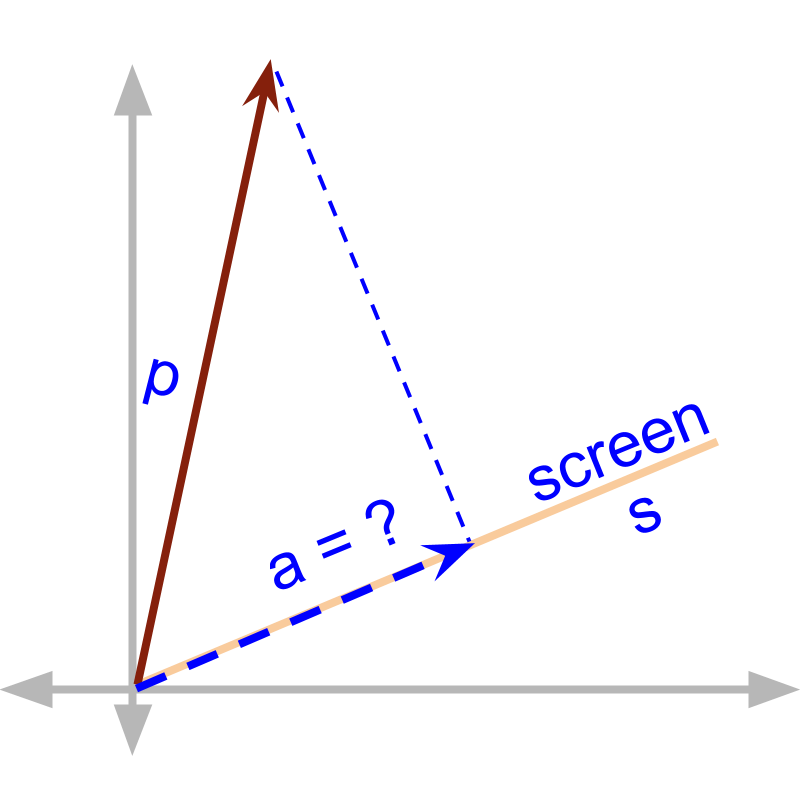maths > vector-algebra

what you'll learn...

Overview

»  By definition, dot product is scalar

»  Some applications may specify an additional direction in the multiplication of components in parallel. In such cases, the direction is combined.

think different

An object by position vector $\stackrel{\to }{p}$$\vec{p}$ casts a shadow on a screen. The screen in given by unit vector $\stackrel{\to }{s}$$\vec{s}$. The shadow is a vector that spans as a ray with a starting and end point.$\stackrel{\to }{p}$$\vec{p}$ and the screen $\stackrel{^}{s}$$\hat{s}$ are given. The length of the shadow is $\stackrel{\to }{p}\cdot \stackrel{^}{s}$$\vec{p} \cdot \hat{s}$ which is a scalar $|p|\mathrm{cos}\theta$$| p | \cos \theta$

The shadow is a vector along the direction of $\stackrel{^}{s}$$\hat{s}$ and the length of the shadow is $\stackrel{\to }{p}\cdot \stackrel{^}{s}$$\vec{p} \cdot \hat{s}$. Combining thest to the shadow is given as $\left(\stackrel{\to }{p}\cdot \stackrel{^}{s}\right)\stackrel{^}{s}$$\left(\vec{p} \cdot \hat{s}\right) \hat{s}$

summary

Projection vector using Dot Product: For a vector $\stackrel{\to }{p}$$\vec{p}$ and a direction given by unit vector $\stackrel{^}{s}$$\hat{s}$, the projection vector of $\stackrel{\to }{p}$$\vec{p}$ in direction $\stackrel{^}{s}$$\hat{s}$ is $\left(\stackrel{\to }{p}\cdot \stackrel{^}{s}\right)\stackrel{^}{s}$$\left(\vec{p} \cdot \hat{s}\right) \hat{s}$.

Vector dot product by definition is a scalar. Depending on the application requirement, a direction can be added to the scalar.

Outline